# Python Math Module

## Python Programming Training Certification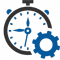Flexible Hours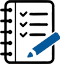100 Assignments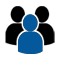Instructor Led online Training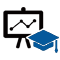50 LMS Access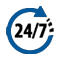24X7 Support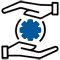100% Skill Level

### Enquire Now

4.9 out of 1000+ Ratings
Best Python Institute for Learning Python Course & Training, Live Project Training in Python with Django, Data Science and AI, Interview Assistance, Expert Coaching Trainers. Python Certification & Interview Assistance! Get free demo now!

### Course Overview

Python is one of the world’s top programming languages used today and Python training has become the most popular training across individuals. Training Basket’s Python Training & Certification course covers basic and advanced Python concepts and how to apply them in real-world applications.Python is a flexible and powerful open-source language that is easy to learn and consists of powerful libraries for data analysis and manipulation. Our Python training course content is curated by experts as per the standard Industry curriculum. The curriculum, coding challenges and real-life problems cover data operations in Python, strings, conditional statements, error handling, shell scripting, web scraping and the commonly used Python web framework Django. Take this Python training and certification course and become job-ready now.### Python Math Module

Python math module is defined as the most famous mathematical functions, which includes trigonometric functions, representation functions, logarithmic functions, etc. Furthermore, it also defines two mathematical constants, i.e., Pie and Euler number, etc.

Pie (n): It is a well-known mathematical constant and defined as the ratio of circumstance to the diameter of a circle. Its value is 3.141592653589793.

Euler’s number(e): It is defined as the base of the natural logarithmic, and its value is 2.718281828459045.

There are different math modules which are given below:

math.log()

This method returns the natural logarithm of a given number. It is calculated to the base e.

Example

• import math
number = 2e-7 # small value of of x
print(‘log(fabs(x), base) is :’, math.log(math.fabs(number), 10))

Output:

• log(fabs(x), base) is : -6.698970004336019

math.log10()

This method returns base 10 logarithm of the given number and called the standard logarithm.

Example

• import math
x=13 # small value of of x
print(‘log10(x) is :’, math.log10(x))

Output:

• log10(x) is : 1.1139433523068367

math.exp()

This method returns a floating-point number after raising e to the given number.

Example

• import math
number = 5e-2 # small value of of x
print(‘The given number (x) is :’, number)
print(‘e^x (using exp() function) is :’, math.exp(number)-1)

Output:

• The given number (x) is : 0.05
e^x (using exp() function) is : 0.05127109637602412

math.pow(x,y)

This method returns the power of the x corresponding to the value of y. If value of x is negative or y is not integer value than it raises a ValueError.

Example

• import math
number = math.pow(10,2)
print(“The power of number:”,number)

Output:

• The power of number: 100.0

math.floor(x)

This method returns the floor value of the x. It returns the less than or equal value to x.

Example:

• import math
number = math.floor(10.25201)
print(“The floor value is:”,number)

Output:

• The floor value is: 10

math.ceil(x)

This method returns the ceil value of the x. It returns the greater than or equal value to x.

• import math
number = math.ceil(10.25201)
print(“The floor value is:”,number)

Output:

• The floor value is: 11

math.fabs(x)

This method returns the absolute value of x.

• import math
number = math.fabs(10.001)
print(“The floor absolute is:”,number)

Output:

• The absolute value is: 10.001

math.factorial()

This method returns the factorial of the given number x. If x is not integral, it raises a ValueError.

Example

• import math
number = math.factorial(7)
print(“The factorial of number:”,number)

Output:

• The factorial of number: 5040

math.modf(x)

This method returns the fractional and integer parts of x. It carries the sign of x is float.

Example

• import math
number = math.modf(44.5)
print(“The modf of number:”,number)

Output:

• The modf of number: (0.5, 44.0)

Python provides the several math modules which can perform the complex task in single-line of code. In this tutorial, we have discussed a few important math modules.

### Recently Trained Students#### Jessica Biel

– Infosys

My instructor had sound Knowledge and used to puts a lot of effort that made the course as simple and easy as possible. I was aiming for with the help of the ZebLearn Online training imparted to me by this organization.#### Richard Harris

– ITC

I got my training from Zeblearn in the Python Certification Training, I would like to say that say he is one of the best trainers. He has not even trained me but also motivated me to explore more and the way he executed the project, in the end, was mind-blowing.

### Candidate’s Journey During Our Training Program#### Expert’s Advice & Selection of Module

Choosing the right type of module for the training is half the battle & Our Team of experts will help & guide you.#### Get Trained

Get Trained & Learn End to End Implementation from our Expert Trainer who are working on the same domain.#### Work on Projects

We Do make our student’s work on multiple case studies , scenario based tasks & projects in order to provide real-time exposure to them.#### Placements

We have a dedicated placement cell in order to provide placement assistance & relevant interviews to our candididates till selection